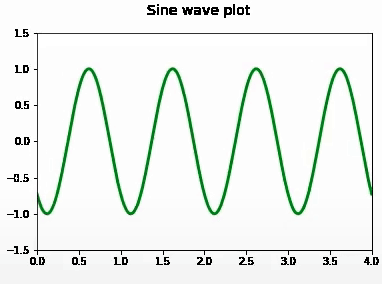# How to save Matplotlib Animation?

• Last Updated : 03 Mar, 2021

In this article, we will learn How to save Matplotlib Animation. The animated graphs made with the help of matplotlib can be saved as videos in Python. As we can create captivating animations using the matplotlib library. If you want to learn to create animations, here is a link to the article to create animations using the matplotlib. In this article, we will learn how to save animation in matplotlib. To save an animation, we can use Animation.save() or Animation.to_html5_video().

Animation.to_html5_video() returns the animation as an HTML5 video tag. It saves the animation as h264 encoded video, which can be directly displayed in the notebook.

Example 1:

## Python3

 `# importing required libraries``from` `matplotlib ``import` `pyplot as plt``import` `numpy as np``import` `matplotlib.animation as animation``from` `IPython ``import` `display`` ` `# initializing a figure``fig ``=` `plt.figure()`` ` `# labeling the x-axis and y-axis``axis ``=` `plt.axes(xlim``=``(``0``, ``4``),  ylim``=``(``-``1.5``, ``1.5``))`` ` `# initializing a line variable``line, ``=` `axis.plot([], [], lw``=``3``)`` ` `def` `animate(frame_number):``    ``x ``=` `np.linspace(``0``, ``4``, ``1000``)`` ` `    ``# plots a sine graph``    ``y ``=` `np.sin(``2` `*` `np.pi ``*` `(x ``-` `0.01` `*` `frame_number))``    ``line.set_data(x, y)``    ``line.set_color(``'green'``)``    ``return` `line,`` ` ` ` `anim ``=` `animation.FuncAnimation(fig, animate, frames``=``100``, ``                               ``interval``=``20``, blit``=``True``)``fig.suptitle(``'Sine wave plot'``, fontsize``=``14``)`` ` `# converting to an html5 video``video ``=` `anim.to_html5_video()`` ` `# embedding for the video``html ``=` `display.HTML(video)`` ` `# draw the animation``display.display(html)``plt.close()`

Output:For using the Animation.save() method to save the animation, we need to provide the writer parameter. Here are few common writers available to write the animation:

PillowWriter: It relies on pillow library. It is preferred when you want to save the animation in gif format.

FFMpegWriter: pipe-based ffmpeg writer.

ImageMagickWriter: pipe-based animated gif.

AVConvWriter: pipe-based avconv writer.

Example 2:

## Python3

 `# importing required libraries``from` `matplotlib ``import` `pyplot as plt``import` `numpy as np``import` `matplotlib.animation as animation``from` `IPython ``import` `display`` ` `# initializing a figure``fig ``=` `plt.figure()`` ` `# labeling the x-axis and y-axis``axis ``=` `plt.axes(xlim``=``(``0``, ``1000``),  ylim``=``(``0``, ``1000``))`` ` `# lists storing x and y values``x, y ``=` `[], []`` ` `line, ``=` `axis.plot(``0``, ``0``)`` ` ` ` `def` `animate(frame_number):``    ``x.append(frame_number)``    ``y.append(frame_number)``    ``line.set_xdata(x)``    ``line.set_ydata(y)``    ``return` `line,`` ` ` ` `anim ``=` `animation.FuncAnimation(fig, animate, frames``=``1000``, ``                               ``interval``=``20``, blit``=``True``)``fig.suptitle(``'Straight Line plot'``, fontsize``=``14``)`` ` `# saving to m4 using ffmpeg writer``writervideo ``=` `animation.FFMpegWriter(fps``=``60``)``anim.save(``'increasingStraightLine.mp4'``, writer``=``writervideo)``plt.close()`

Output:My Personal Notes arrow_drop_up